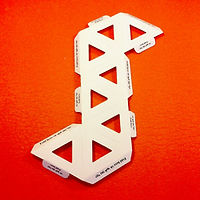OCTAHEDRON

What you need

1/2 octafold

(2 pairs of triangle segments)

The folding process:

1)  Prepare a single octafold unit

2) Fold into the octahedron by pulling head to tail in a spiraling movement.

!

The octahedron is slightly more complex than the tetrahedron. The square pyramids built by the Egyptian Pharaohs are each half an octahedron. The octahedron is one of the five Plato solids. Plato ascribed the element of air to this shape. There are 11 ways  (nets) to fold an octahedron from two-dimensional nets. The octafold building unit is one of these nets.?

Question

What natural element tends to arrange itself as an octahedron?

Carbon. As carbon crystallizes into a diamond, it often crystallizes in the shape of the octahedron.?

Question

What shape would we get if we attach a tetrahedron to each of the eight faces of the octahedron?